mt_plot can be used for plotting a number of individual trajectories. mt_plot_aggregate can be used for plotting aggregated trajectories. The color and linetype can be varied depending on a set of condition variables using the color and linetype arguments. If the x and y arguments are varied, this function can also be used for plotting velocity and acceleration profiles.

mt_plot(
data,
use = "trajectories",
use2 = "data",
x = "xpos",
y = "ypos",
color = NULL,
linetype = NULL,
alpha = NA,
size = 0.5,
facet_row = NULL,
facet_col = NULL,
wrap_var = NULL,
wrap_ncol = NULL,
points = FALSE,
return_type = "plot",
mt_id = "mt_id",
only_ggplot = NULL,
...
)

mt_plot_aggregate(
data,
use = "trajectories",
use2 = "data",
x = "xpos",
y = "ypos",
color = NULL,
linetype = NULL,
alpha = NA,
size = 0.5,
facet_row = NULL,
facet_col = NULL,
wrap_var = NULL,
wrap_ncol = NULL,
points = FALSE,
return_type = "plot",
subject_id = NULL,
only_ggplot = NULL,
...
)

## Arguments

data

a mousetrap data object created using one of the mt_import functions (see mt_example for details). Alternatively, a trajectory array can be provided directly (in this case use will be ignored).

use

a character string specifying which trajectories should be plotted. The corresponding trajectories are selected from data using data[[use]]. Usually, this value corresponds to either "trajectories", "tn_trajectories" or "av_trajectories", depending on whether the raw, time-normalized or averaged trajectories should be plotted.

use2

a character string specifying where the data that contain the variables used for determining the color and linetype can be found (in case these arguments are specified). Defaults to "data" as data[["data"]] usually contains all non mouse-tracking trial data.

x

a character string specifying which dimension in the trajectory array should be displayed on the x-axis (defaults to xpos).

y

a character string specifying which dimension in the trajectory array should be displayed on the y-axis (defaults to ypos).

color

an optional character string specifying which variable in data[[use2]] should be used for coloring the trajectories.

linetype

an optional character string specifying which variable in data[[use2]] should be used for varying the linetype of the trajectories.

alpha

an optional numeric value between 0 and 1 that can be used to make the plotted lines (and points) semitransparent.

size

an optional numeric value that can be used to vary the width of the plotted trajectory lines.

facet_row

an optional character string specifying a variable in data[[use2]] that should be used for (row-wise) faceting.

facet_col

an optional character string specifying a variable in data[[use2]] that should be used for (column-wise) faceting.

wrap_var

an optional character string specifying variable(s) in data[[use2]] that should be used for wrapping.

wrap_ncol

an optional integer specifying the number of columns if wrapping is used.

points

logical. If TRUE, points will be added to the plot using geom_point.

return_type

a character string specifying which type of object should be returned. If "plot" (the default), a new ggplot is created and the trajectories are plotted using geom_path. If "mapping", only the ggplot object containing the mapping but without any geoms is returned. If "geoms", only the geoms are returned, which allows adding the plotted trajectories to an existing ggplot.

mt_id

a character string specifying the internal label used for the trial identifier (passed on to the group aesthetic). Only relevant for mt_plot.

only_ggplot

Deprecated. Please use return_type instead.

...

additional arguments passed on to mt_reshape (such as subset).

subject_id

a character string specifying which column contains the subject identifier. Only relevant for mt_plot_aggregate. If specified, aggregation will be performed within subjects first. Note that aggregation will be performed separately for each level, including all subjects for whom data are available.

## Details

mt_plot internally uses mt_reshape for reshaping trajectories into a long format. Next, it creates a ggplot object using the ggplot function of the ggplot2 package. The aes mappings are taken from the function arguments for x, y etc.; in addition, the group mapping is set to the internal trial identifier (by default called "mt_id").

If return_type == "plot" (the default), a new ggplot is created and the trajectories are plotted using the geom_path function of the ggplot2 package. If return_type == "mapping", the ggplot object is returned without layers, which can be used to further customize the plot (see Examples). If return_type == "geoms", only the geoms are returned, which allows adding the plotted trajectories to an existing ggplot (e.g., adding aggregate trajectories on top of the individual trajectories, see Examples).

mt_plot_aggregate works similarly, but uses mt_aggregate for reshaping and aggregating trajectories prior to plotting.

Please note that this function is intended as a quick and easy solution for visualizing mouse trajectories. For additional flexibility, we recommend that mt_reshape or mt_aggregate be used in conjunction with ggplot to create custom visualizations.

## Functions

• mt_plot(): Plot individual trajectory data

• mt_plot_aggregate(): Plot aggregated trajectory data

mt_plot_riverbed for plotting the relative frequency of a selected variable across time.

mt_plot_per_trajectory for individually plotting all trajectories as individual pdf files.

## Author

Pascal J. Kieslich

Felix Henninger

## Examples

## Plot individual example trajectories

# Time-normalize trajectories
mt_example <- mt_time_normalize(mt_example)

# Plot all time-normalized trajectories
# varying the color depending on the condition
mt_plot(mt_example, use="tn_trajectories",
color="Condition")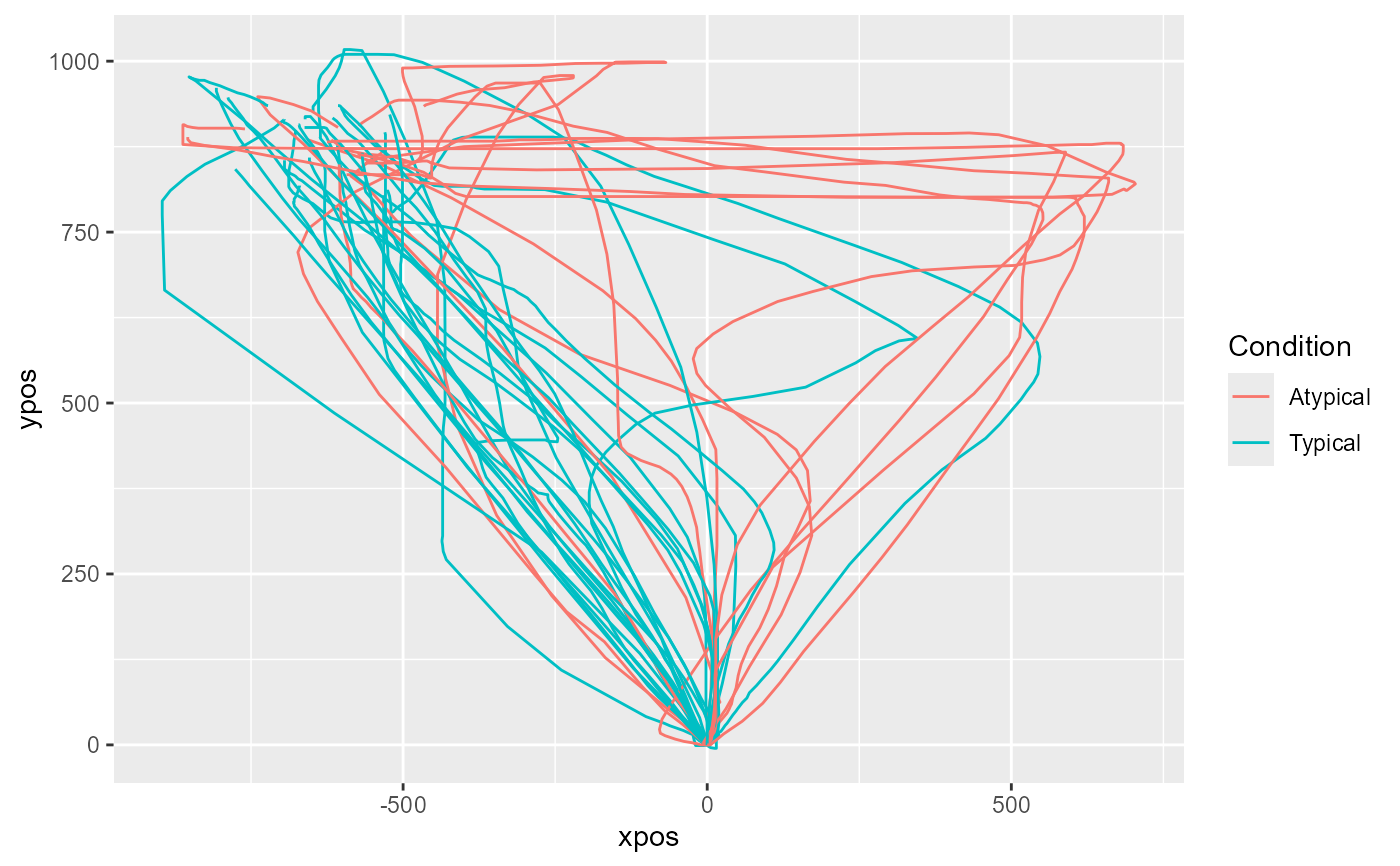# ... setting alpha < 1 for semi-transparency
mt_plot(mt_example, use="tn_trajectories",
color="Condition", alpha=.2)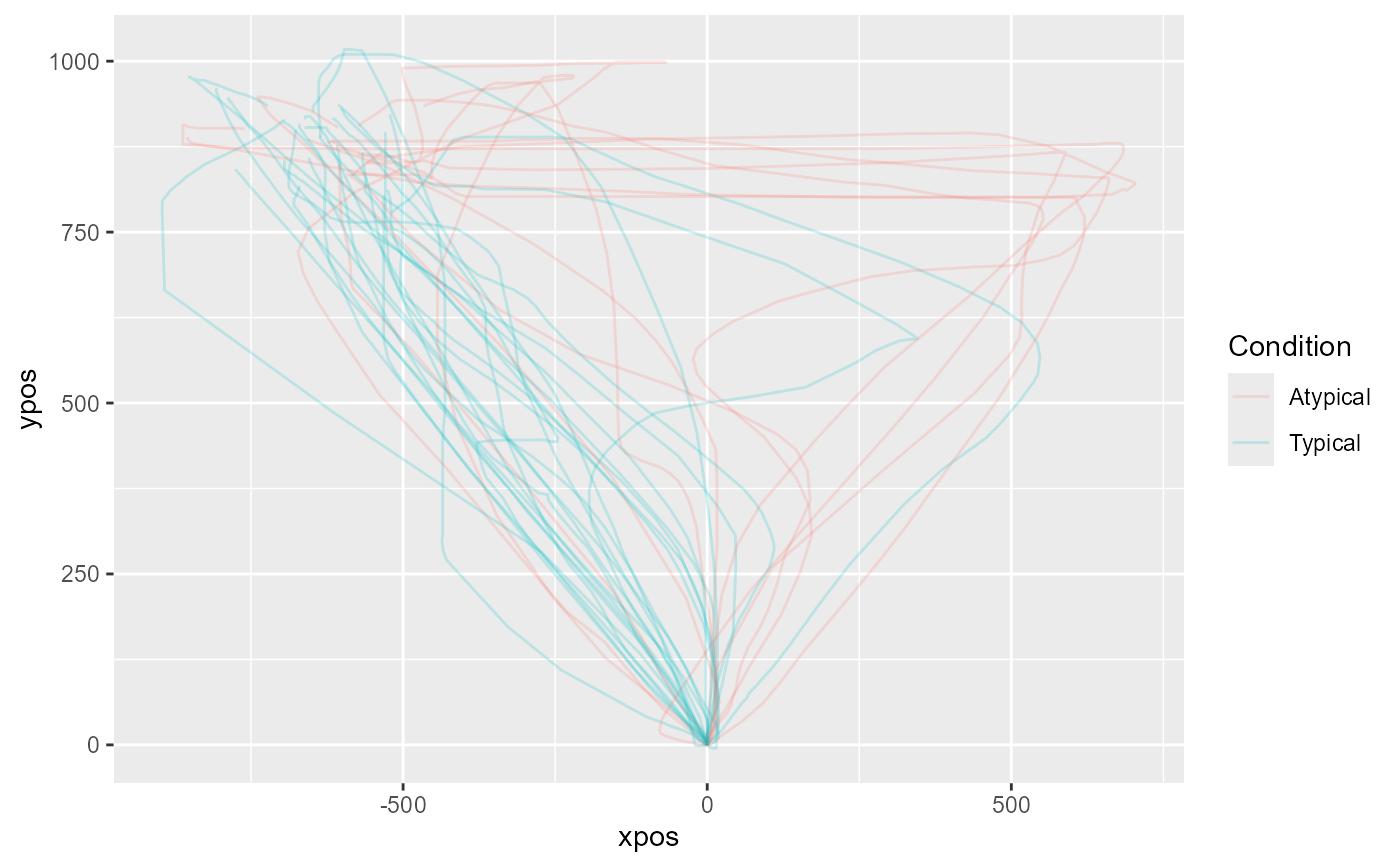# ... with custom colors
mt_plot(mt_example, use="tn_trajectories",
color="Condition") +
ggplot2::scale_color_brewer(type="qual")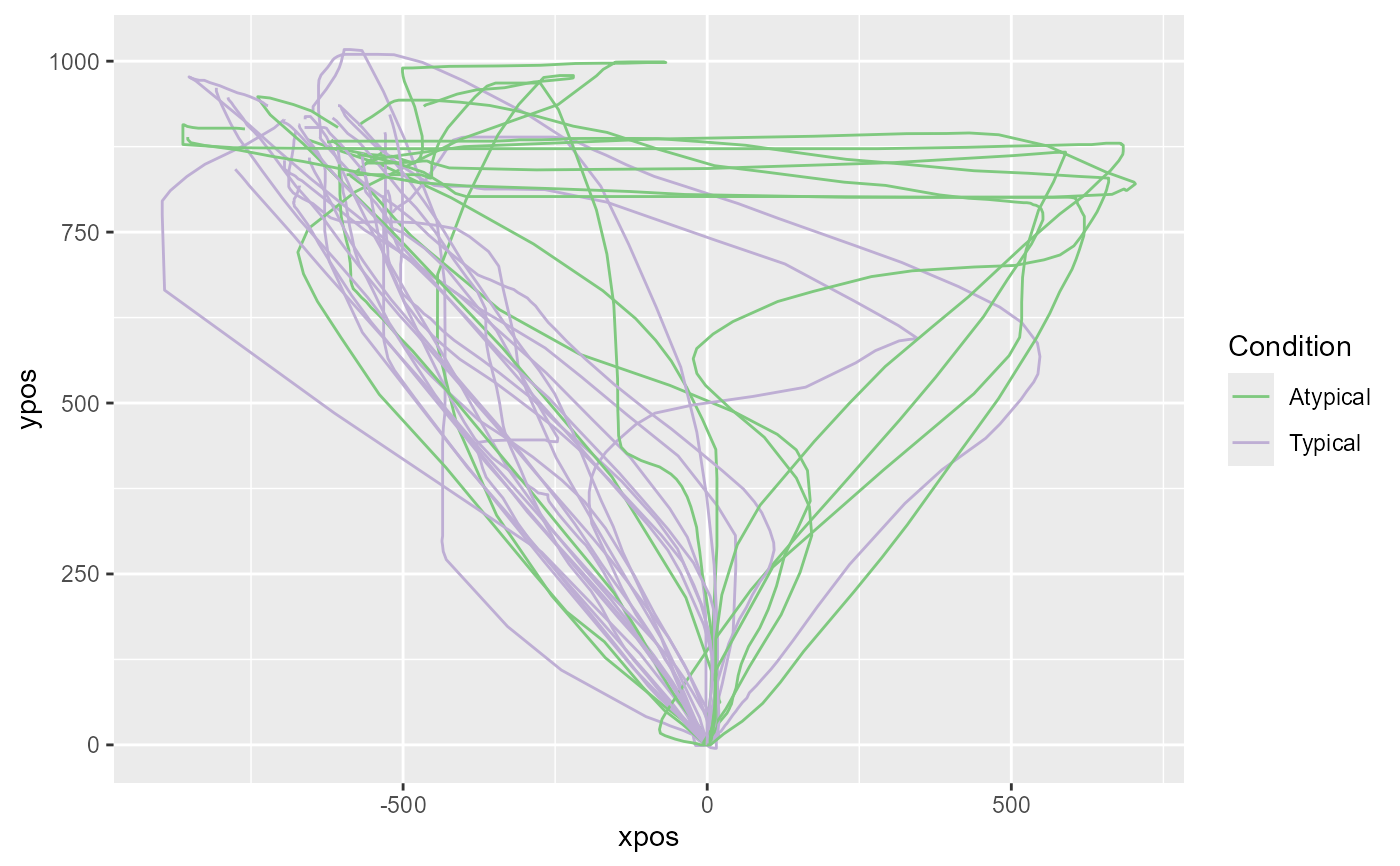# Create separate plots per Condition
mt_plot(mt_example, use="tn_trajectories",
facet_col="Condition")# Create customized plot by setting the return_type option to "mapping"
# to setup an empty plot. In a next step, a geom is added.
# In this example, only points are plotted.
mt_plot(mt_example, use="tn_trajectories",
color="Condition", return_type="mapping") +
ggplot2::geom_point()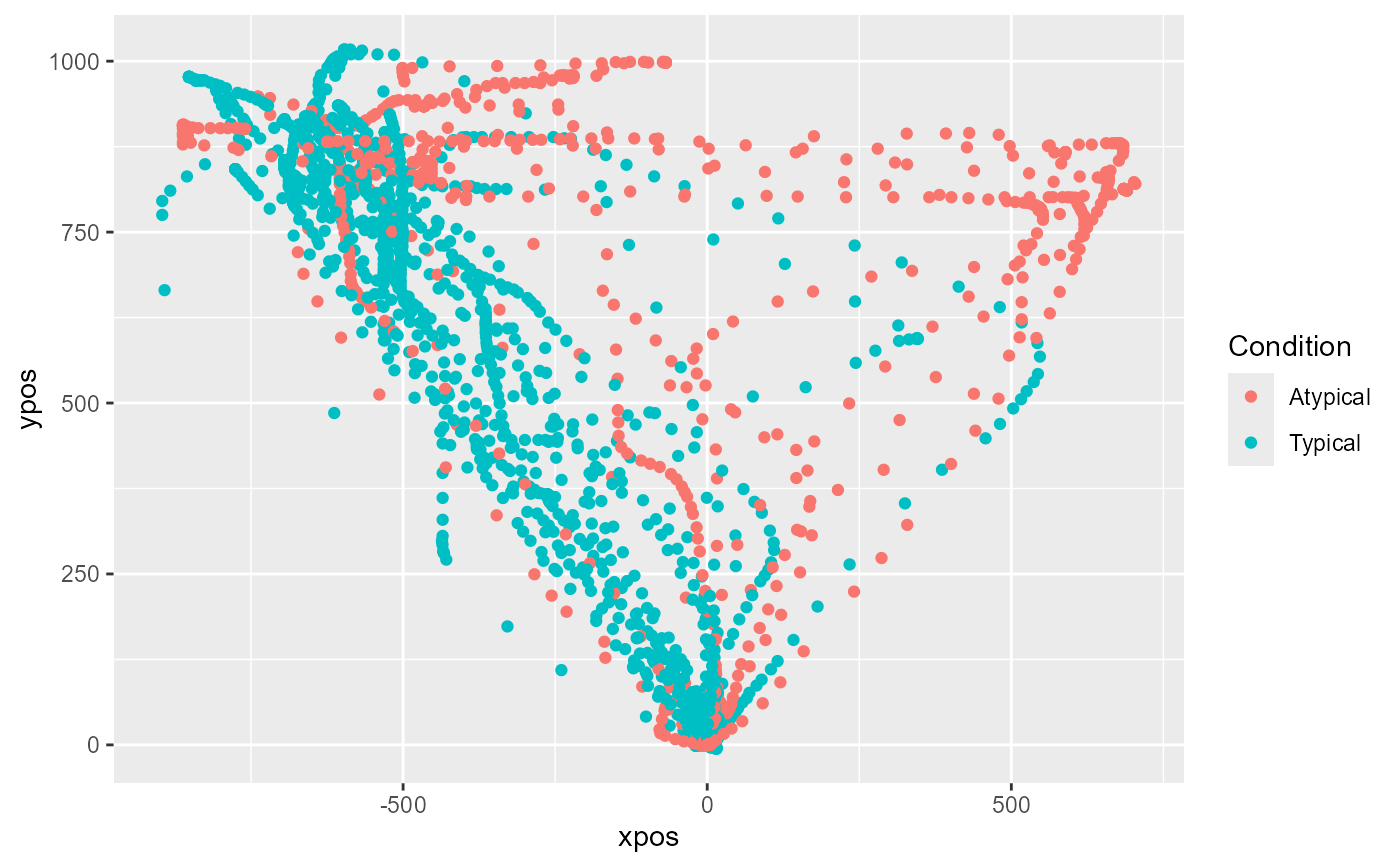# Plot velocity profiles based on the averaged trajectories
# varying the color depending on the condition
mt_example <- mt_derivatives(mt_example)
mt_example <- mt_average(mt_example, interval_size=100)
mt_plot(mt_example, use="av_trajectories",
x="timestamps", y="vel", color="Condition")## Plot aggregate trajectories for KH2017 data

# Time-normalize trajectories
KH2017 <- mt_time_normalize(KH2017)

# Plot aggregated time-normalized trajectories per condition
mt_plot_aggregate(KH2017, use="tn_trajectories",
color="Condition")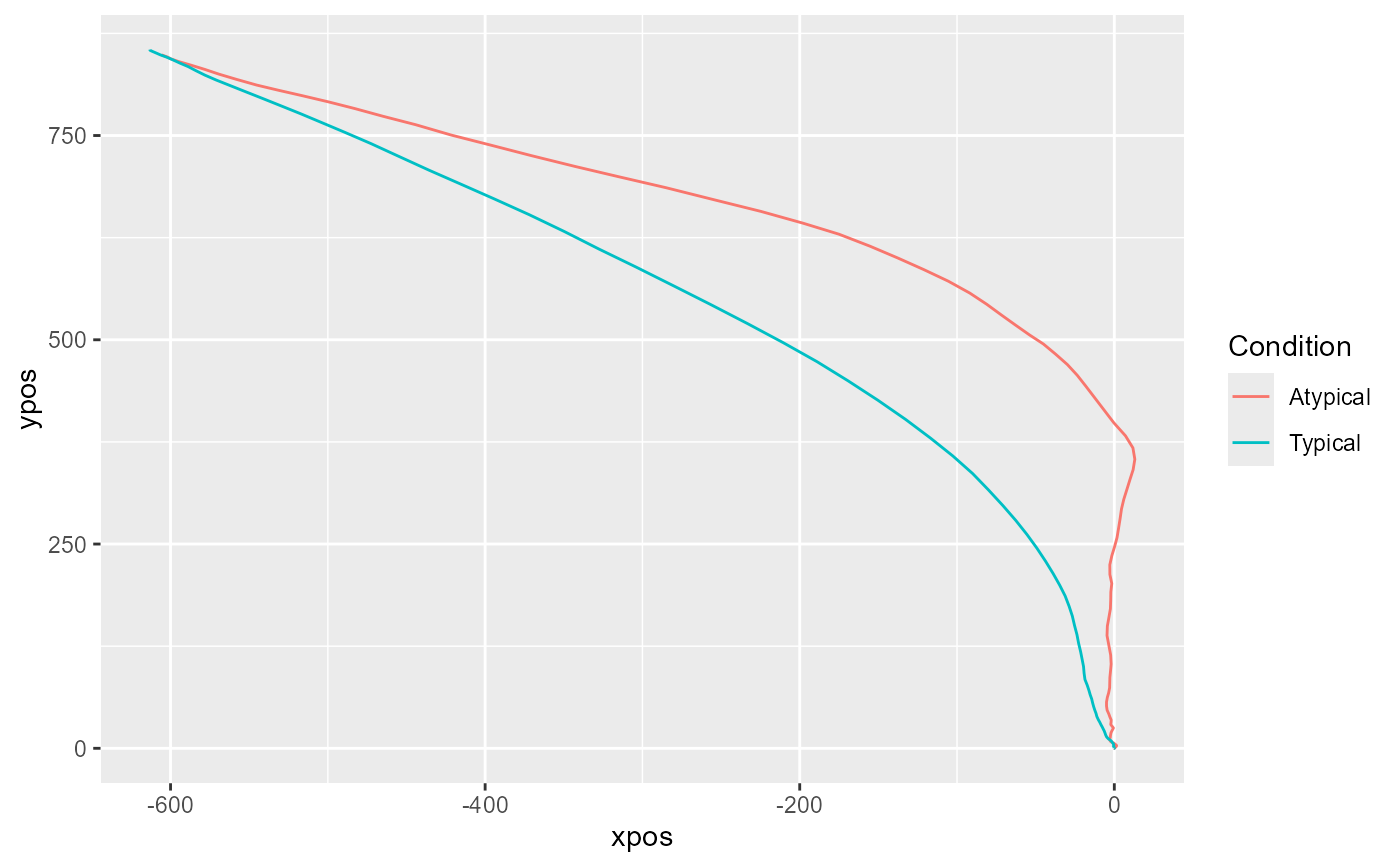# ... first aggregating trajectories within subjects
mt_plot_aggregate(KH2017, use="tn_trajectories",
color="Condition", subject_id="subject_nr")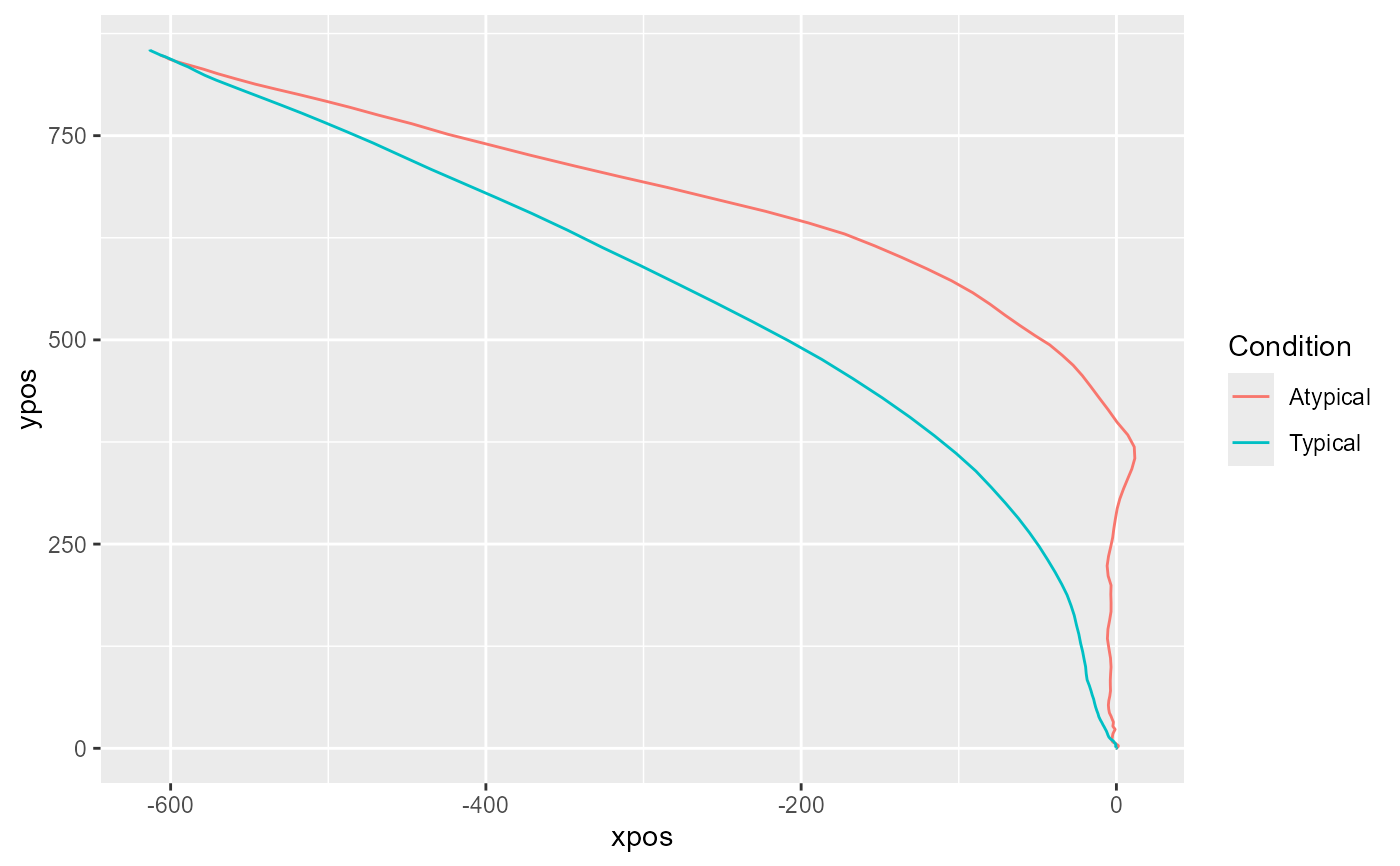# ... adding points for each position to the plot
mt_plot_aggregate(KH2017, use="tn_trajectories",
color="Condition", points=TRUE)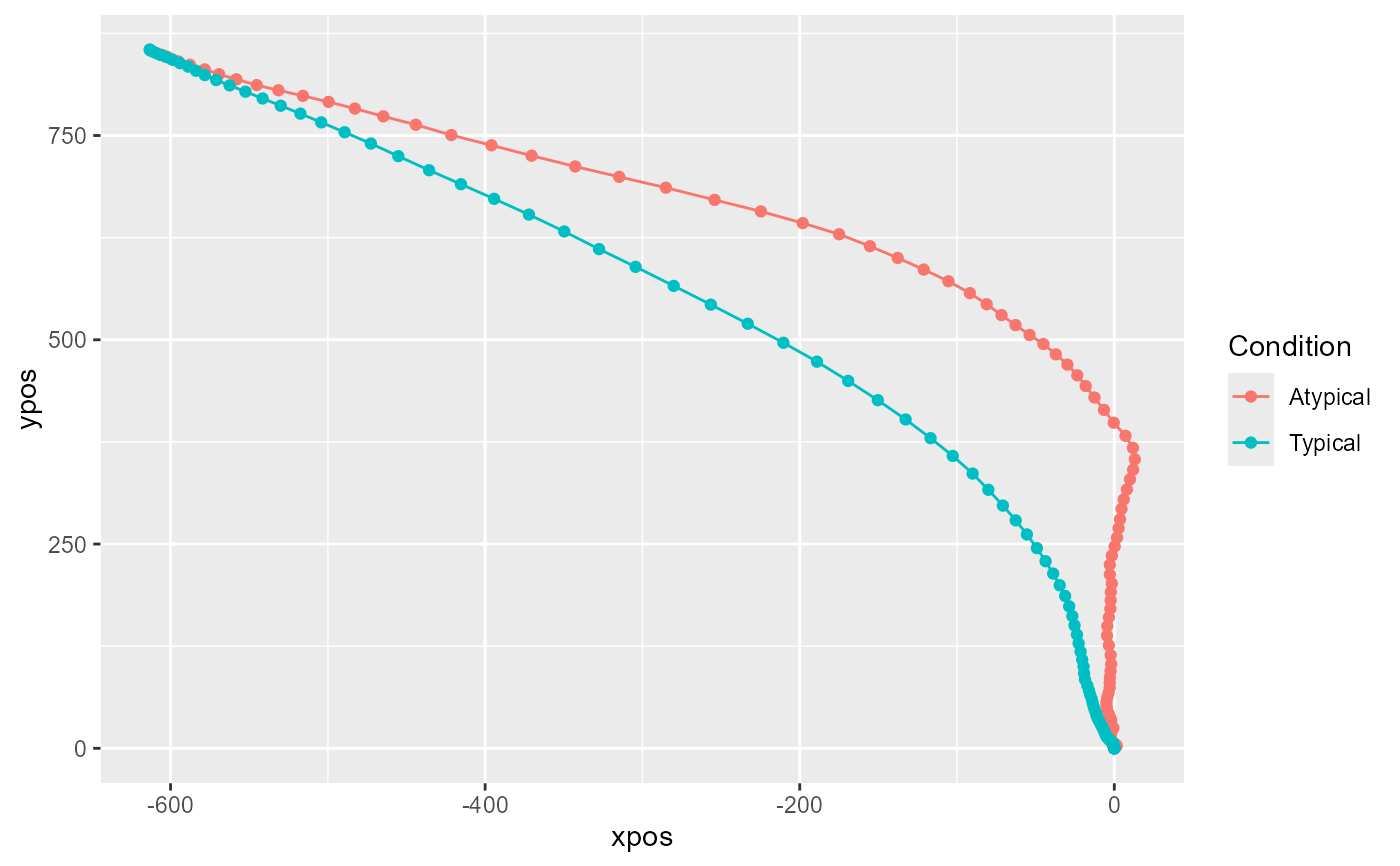if (FALSE) {

# Create combined plot of individual and aggregate trajectories
# by first plotting the individual trajectories using mt_plot.
# In a next step, the aggregate trajectories are added using the
# mt_plot_aggregate function with the return_type argument set to "geom".
mt_plot(KH2017, use="tn_trajectories", color="Condition", alpha=.05) +
mt_plot_aggregate(KH2017, use="tn_trajectories",
color="Condition", return_type="geom", size=2)
}